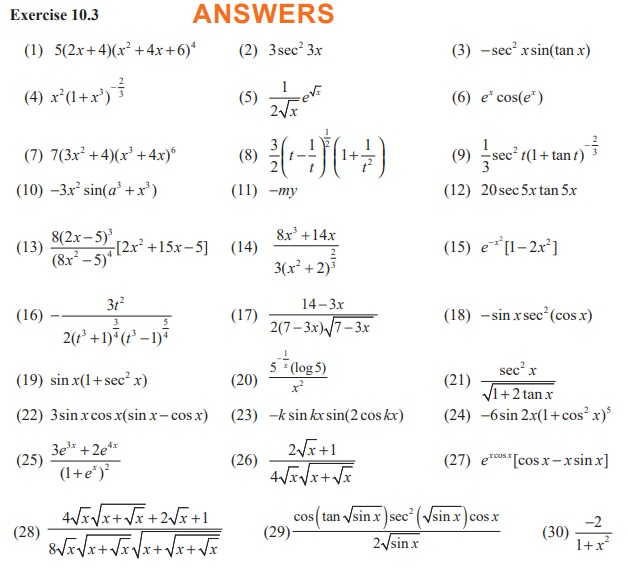Home | | Maths 11th std | Examples on Chain Rule (Differentiation Rules)

# Examples on Chain Rule (Differentiation Rules)

Differentiation Rules - Differential Calculus

Examples on Chain Rule

Example 10.8

Find F′(x) if F (x) = √[x2 +1]

Solution

Take u = g(x) = x2 + 1 and f (u) =√u

F (x) = ( fog)(x) = f (g(x))Example 10.9

Differentiate : (i) y = sin(x2) (ii) y = sin2x

Solution

(i) The outer function is the sine function and the inner function is the squaring function.

Let u = x2

That is, y = sin u.EXERCISE 10.3

Differentiate the following :Study Material, Lecturing Notes, Assignment, Reference, Wiki description explanation, brief detail
11th Mathematics : UNIT 10 : Differential Calculus: Differentiability and Methods of Differentiation : Examples on Chain Rule (Differentiation Rules) |